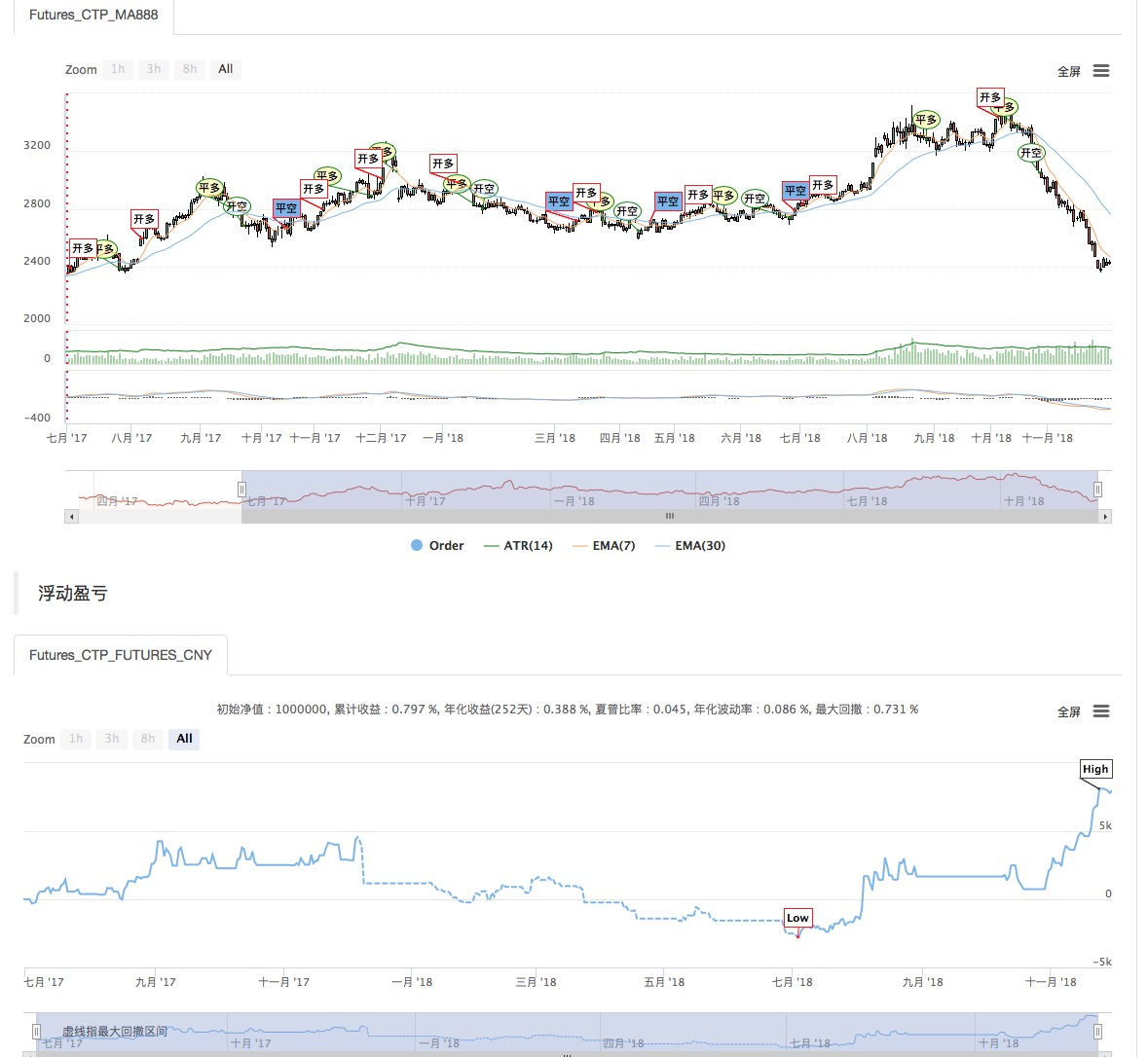# 三轨道波动率策略

Author: , Created: 2019-07-22 10:18:43, Updated: 2019-07-23 10:43:21

``````// 确定CN
VOLAT:=STD(C,N);   // VOLAT（波动率）:M周期收盘价的标准差
VOLATCHANGE:=(VOLAT-REF(VOLAT,1))/VOLAT;    // 2个VOLAT的变化率
N1:=(1+VOLATCHANGE)*MINN;                   // VOLATCHANGE ： 波动率变化
N2:=INTPART(N1);                            // 取整
N3:=MIN(N2,MAXN);                           // 确认CN不大于60
CN:=MAX(N3,MINN);                           // 确认CN不小于20
MIDTR^^MA(C,CN);                            // 确定MIDTR
UPTR^^MIDTR+2*STD(C,CN);                    // 确定UPTR
DOWNTR^^MIDTR-2*STD(C,CN);                  // 确定DOWNTR
HPOINT^^HV(H,CN),DOT,COLORRED;   // 计算前一周期CN周期内最高价的最大值。
LPOINT^^LV(L,CN),DOT,COLORBLUE;  // 计算前一周期CN周期内最低价的最小值。

// 开仓
L<=LPOINT AND L<DOWNTR AND BARPOS>MINN,SK(AMOUNT);  //当最低价小于DOWNTR和低点，且K线位置大于60，收盘价卖开
H>=HPOINT AND H>UPTR AND BARPOS>MINN,BK(AMOUNT);    //当最高价大于UPTR和高点，且K线位置大于60，收盘价买开

// 启动止损
C>=SKPRICE*(1+STOPRANGE*0.001),BP(SKVOL);
C<=BKPRICE*(1-STOPRANGE*0.001),SP(BKVOL);

// 平仓
C<MIDTR,SP(BKVOL); // 当收盘价小于MIDTR，收盘价卖平
C>MIDTR,BP(SKVOL); // 当收盘价大于MIDTR，收盘价买平

// 动态止损
REF(BKHIGH,1)>BKPRICE*(1+2*0.001*STOPRANGE) AND C<HV(C,BARSBK)*(1-STOPRANGE*0.001),SP(BKVOL);  // 买开后最高价大于买开价*(1+2*0.001*STOPRANGE)，且收盘价小于买开后最高收盘价*(1-STOPRANGE*0.001)，收盘价卖平
``````

``````MIDTR^^MA(C,CN); // 确定MIDTR
UPTR^^MIDTR+2STD(C,CN); // 确定UPTR
DOWNTR^^MIDTR-2STD(C,CN); // 确定DOWNTR
HPOINT^^HV(H,CN),DOT,COLORRED; // 计算前一周期CN周期内最高价的最大值。
LPOINT^^LV(L,CN),DOT,COLORBLUE; // 计算前一周期CN周期内最低价的最小值。
``````More